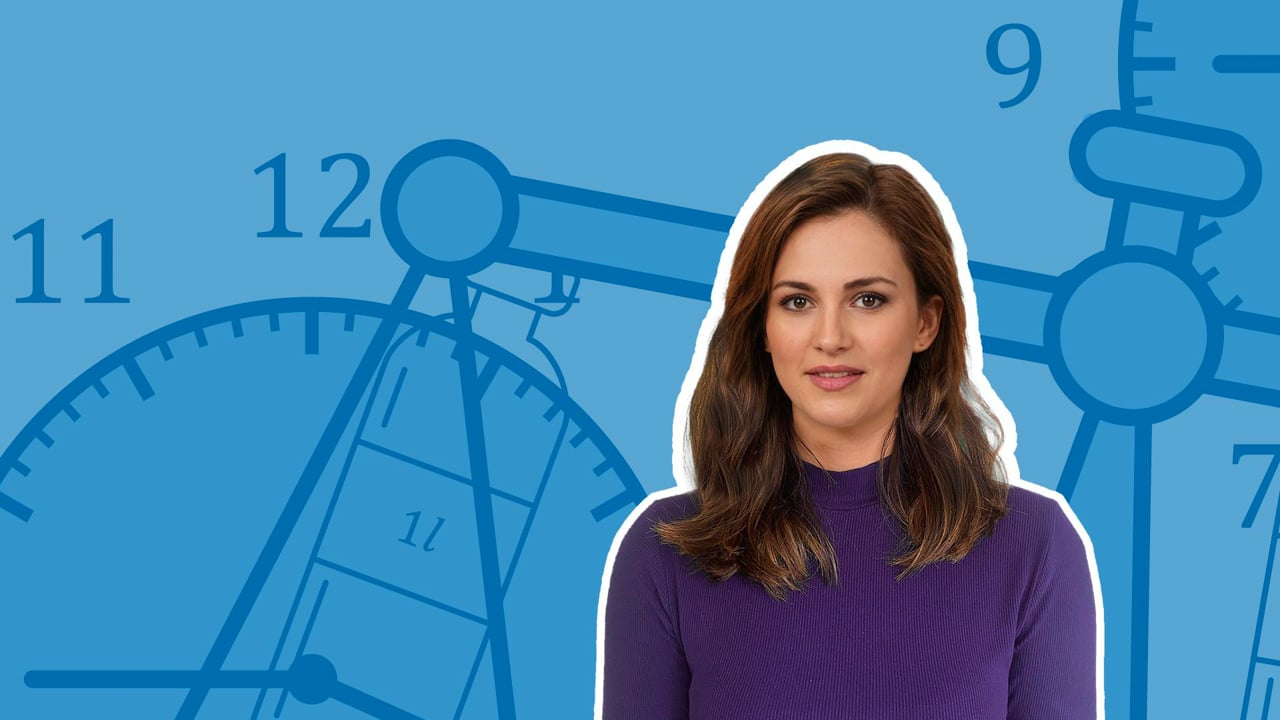Chapter OverviewMaths

Number and place value

Multiplication and division

Fractions

Measurement

Geometry - properties of shapes

Geometry - position and direction

Statistics

Ratio and proportion

Algebra

Maths

# Calculate and convert between units of measure0%

Summary

# Calculate and convert between units of measure

## ​​In a nutshell

By converting between units of measures, measurements can be presented in their most simple forms. These conversions will often involve handling decimals and units of length, mass and volume.

## Conversion rates

The conversion rates you will have to know are listed below.

$1km = 1000m$​​

$1m = 100cm$

$1cm = 10mm$​​​

$1 l = 1000ml$​​

$1kg=1000g$​​

## Converting between units of measures

Use conversion rates to calculate and simplify measurements into a more presentable format.

##### Example 1

What is $0.654kg$​ in grams?

Identify the conversion rate between kilograms and grams.

$1kg = 1000g$​​

To convert kilograms to grams, multiply by $1000$.

$0.654 \times 1000 = 654$​​

Therfore,

$\underline{ 0.654kg = 654 \ grams.}$

##### Example 2

Rewrite $8000mm$ in metres.

First, convert millimetres to centimetres using the conversion rate:

$1cm =10mm$

To convert millimetres to centimetres, divide by $10$.

$8000 \div 10 = 800cm$

Next, use the conversion rate for centimetres to metres.

$1m = 100cm$

To convert centimetres to metres, divide by $100$​.

$800 \div 100 = 8m$

Therefore,

$\underline{8000mm = 8 \ metres. }$

Note: To convert directly from millimetres to metres, divide by $1000$.

## Want to find out more? Check out these other lessons!

FAQs

• Question: How do you convert millimetres to metres?

Answer: To convert directly from millimetres to metres divide by 1000.

• Question: How do you convert millimetres to centimetres?

Answer: To convert millimetres to centimetres divide by 10.

• Question: What is the conversion rate for kilograms to grams?

Answer: 1kg is equal to 1000 grams.

Theory

Exercises